• 循环神经网络应用举例

千次阅读 2017-10-09 01:29:21
我们首先把词依次输入到循环神经网络中，每输入一个词，循环神经网络就输出截止到目前为止，下一个最可能的词。例如，当我们依次输入： 我 昨天 上学 迟到 了 神经网络的输出如下图所示： 其中，s和e...

RNN的应用举例——基于RNN的语言模型

现在，我们介绍一下基于RNN语言模型。我们首先把词依次输入到循环神经网络中，每输入一个词，循环神经网络就输出截止到目前为止，下一个最可能的词。例如，当我们依次输入：

我 昨天 上学 迟到 了

神经网络的输出如下图所示：其中，s和e是两个特殊的词，分别表示一个序列的开始和结束。

向量化

我们知道，神经网络的输入和输出都是向量，为了让语言模型能够被神经网络处理，我们必须把词表达为向量的形式，这样神经网络才能处理它。

神经网络的输入是，我们可以用下面的步骤对输入进行向量化

1. 建立一个包含所有词的词典，每个词在词典里面有一个唯一的编号。
2. 任意一个词都可以用一个N维的one-hot向量来表示。其中，N是词典中包含的词的个数。假设一个词在词典中的编号是i，v是表示这个词的向量， vj 是向量的第j个元素，则：

vj={1j=i0ji(76)

上面这个公式的含义，可以用下面的图来直观的表示：使用这种向量化方法，我们就得到了一个高维、稀疏的向量（稀疏是指绝大部分元素的值都是0）。处理这样的向量会导致我们的神经网络有很多的参数，带来庞大的计算量。因此，往往会需要使用一些降维方法，将高维的稀疏向量转变为低维的稠密向量。不过这个话题我们就不再这篇文章中讨论了。

语言模型要求的输出是下一个最可能的词，我们可以让循环神经网络计算计算词典中每个词是下一个词的概率，这样，概率最大的词就是下一个最可能的词。因此，神经网络的输出向量也是一个N维向量，向量中的每个元素对应着词典中相应的词是下一个词的概率。如下图所示：Softmax层

前面提到，语言模型是对下一个词出现的概率进行建模。那么，怎样让神经网络输出概率呢？方法就是用softmax层作为神经网络的输出层。

我们先来看一下softmax函数的定义：

g(zi)=ezikezk

这个公式看起来可能很晕，我们举一个例子。Softmax层如下图所示：从上图我们可以看到，softmax layer的输入是一个向量，输出也是一个向量，两个向量的维度是一样的（在这个例子里面是4）。输入向量x=[1 2 3 4]经过softmax层之后，经过上面的softmax函数计算，转变为输出向量y=[0.03 0.09 0.24 0.64]。计算过程为：

y1y2y3y4=ex1kexk=e1e1+e2+e3+e4=0.03=e2e1+e2+e3+e4=0.09=e3e1+e2+e3+e4=0.24=e4e1+e2+e3+e4=0.64(77)(78)(79)(80)(81)(82)(83)(84)(85)

我们来看看输出向量y的特征：

1. 每一项为取值为0-1之间的正数；
2. 所有项的总和是1。

我们不难发现，这些特征和概率的特征是一样的，因此我们可以把它们看做是概率。对于语言模型来说，我们可以认为模型预测下一个词是词典中第一个词的概率是0.03，是词典中第二个词的概率是0.09，以此类推。

语言模型的训练

可以使用监督学习的方法对语言模型进行训练，首先，需要准备训练数据集。接下来，我们介绍怎样把语料

我 昨天 上学 迟到 了

转换成语言模型的训练数据集。

首先，我们获取输入-标签对：

输入 标签
s
昨天
昨天 上学
上学 迟到
迟到
e

然后，使用前面介绍过的向量化方法，对输入x和标签y进行向量化。这里面有意思的是，对标签y进行向量化，其结果也是一个one-hot向量。例如，我们对标签『我』进行向量化，得到的向量中，只有第2019个元素的值是1，其他位置的元素的值都是0。它的含义就是下一个词是『我』的概率是1，是其它词的概率都是0。

最后，我们使用交叉熵误差函数作为优化目标，对模型进行优化。

在实际工程中，我们可以使用大量的语料来对模型进行训练，获取训练数据和训练的方法都是相同的。

交叉熵误差

一般来说，当神经网络的输出层是softmax层时，对应的误差函数E通常选择交叉熵误差函数，其定义如下：

L(y,o)=1NnNynlogon

在上式中，N是训练样本的个数，向量 yn 是样本的标记，向量 on 是网络的输出。标记 yn 是一个one-hot向量，例如 y1=[1,0,0,0] ，如果网络的输出 o=[0.03,0.09,0.24,0.64] ，那么，交叉熵误差是（假设只有一个训练样本，即N=1）：

L=1NnNynlogon=y1logo1=(1log0.03+0log0.09+0log0.24+0log0.64)=3.51(86)(87)(88)(89)

我们当然可以选择其他函数作为我们的误差函数，比如最小平方误差函数(MSE)。不过对概率进行建模时，选择交叉熵误差函数更make sense。

展开全文深度学习 数据 机器学习 神经网络 算法
• 本例包含reddit论坛数据集，使用rnn对论坛留言进行情感分类。是rnn入门的简单易学教程。
• 循环神经网络应用案例

千次阅读 2018-10-15 22:46:33
# 循环神经网路的截断长度 TRAINING_STEPS = 3000 # 循环轮数 BATCH_SIZE = 32 # batch大小 TRAINING_EXAMPLES = 10000 # 训练数据的个数 TESTING_EXAMPLES = 1000 # 测试数据的个数 ...

tensorflow的编程堆栈示意图：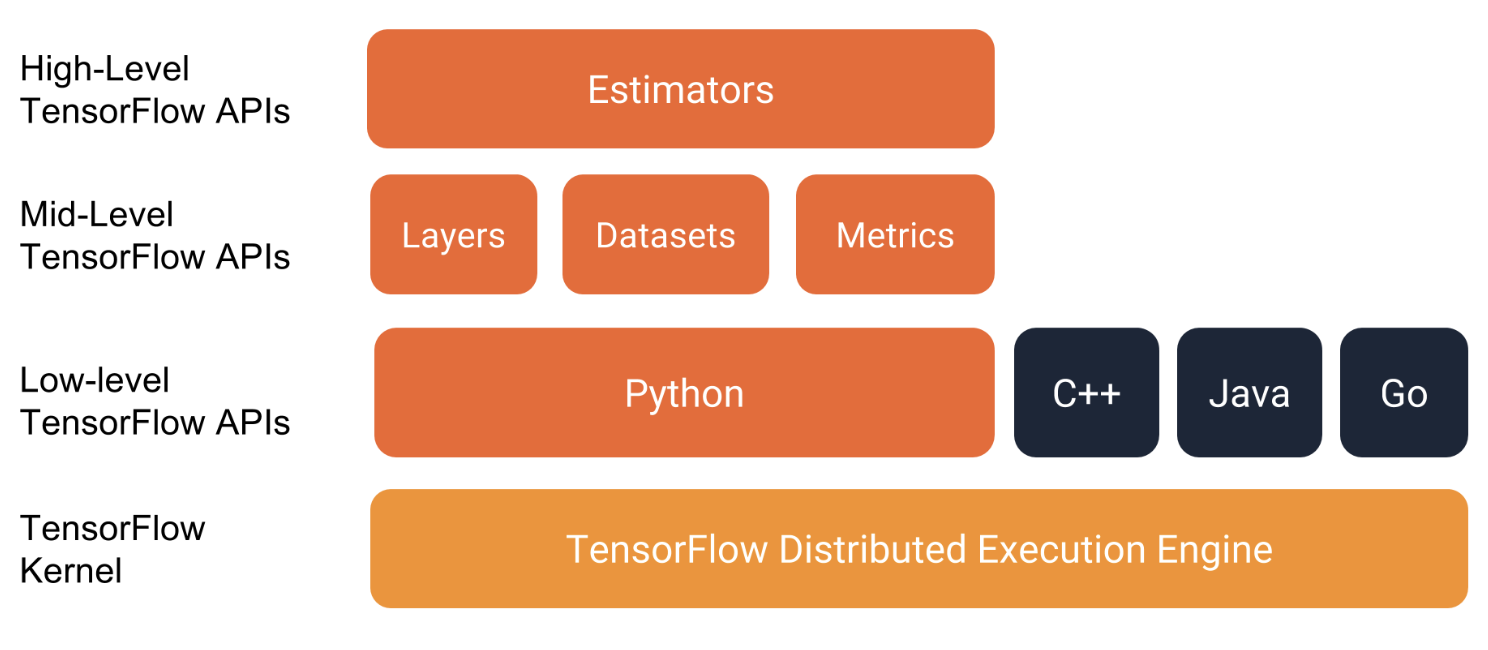强烈建议使用以下API编写TensorFlow程序：

• 评估器Estimators，代表一个完整的模型。 Estimator API提供方法来训练模型，判断模型的准确性并生成预测。
• 训练集Datasets，它构建了一个数据输入管道。Datasets API具有加载和操作数据的方法，并将其输入到你的模型中。Datasets API与Estimators API良好地协作。

1. 基于TFlearn的iris分类

下面介绍利用tensorflow的一个高层封装TFlearn。
iris数据集需要通过4个特征来分辨三种类型的植物，iris数据集中总共包含了150个样本，下面是TFlearn解决iris分类问题：
数据集

鸢尾花数据集包含四个特征和一个标签。这四个特征确定了单个鸢尾花的以下植物学特征：

• 萼片长度
• 萼片宽度
• 花瓣长度
• 花瓣宽度

下表显示了数据集中的三个示例：

萼片长度萼片宽度花瓣长度花瓣宽度种类（标签）
5.13.31.70.50（Setosa）
5.02.33.31.01（Versicolor）
6.42.85.62.22（virginica）

完整代码：

from sklearn import model_selection
from sklearn import datasets
from sklearn import metrics
import tensorflow as tf
import numpy as np
from tensorflow.contrib.learn.python.learn.estimators.estimator import SKCompat

# 导入TFlearn
learn = tf.contrib.learn

# 自定义softmax回归模型：在给定输入的训练集、训练集标签，返回在这些输入上的预测值、损失值以及训练步骤
def my_model(features, target):
# 将预测目标转化为one-hot编码的形式，共有三个类别，所以向量长度为3
target = tf.one_hot(target, 3, 1, 0)
# 计算预测值及损失函数：封装了一个单层全连接的神经网络
logits = tf.contrib.layers.fully_connected(features, 3, tf.nn.softmax)
loss = tf.losses.softmax_cross_entropy(target, logits)

# 创建模型的优化器，并优化步骤
train_op = tf.contrib.layers.optimize_loss(
loss,                                    # 损失函数
tf.contrib.framework.get_global_step(),  # 获取训练步骤并在训练时更新
learning_rate=0.01)                      # 定义学习率

# 返回给定数据集上的预测结果、损失值以及优化步骤
return tf.arg_max(logits, 1), loss, train_op

# 加载iris数据集，并划分为训练集和测试集
x_train, x_test, y_train, y_test = model_selection.train_test_split(
iris.data, iris.target, test_size=0.2, random_state=0)

#将数据转化成TensorFlow要求的float32格式
x_train, x_test = map(np.float32, [x_train, x_test])

# 封装和训练模型，输出准确率
classifier = SKCompat(learn.Estimator(model_fn=my_model, model_dir="Models/model_1"))
classifier.fit(x_train, y_train, steps=100)

# 预测
y_predicted = [i for i in classifier.predict(x_test)]
# 计算准确度
score = metrics.accuracy_score(y_test, y_predicted)

print('Accuracy: %.2f%%' % (score * 100))

运行结果：

INFO:tensorflow:Using default config.
INFO:tensorflow:Using config: {'_task_type': None, '_task_id': 0, '_cluster_spec': <tensorflow.python.training.server_lib.ClusterSpec object at 0x12a8484e0>, '_master': '', '_num_ps_replicas': 0, '_num_worker_replicas': 0, '_environment': 'local', '_is_chief': True, '_evaluation_master': '', '_train_distribute': None, '_device_fn': None, '_tf_config': gpu_options {
per_process_gpu_memory_fraction: 1.0
}
, '_tf_random_seed': None, '_save_summary_steps': 100, '_save_checkpoints_secs': 600, '_log_step_count_steps': 100, '_session_config': None, '_save_checkpoints_steps': None, '_keep_checkpoint_max': 5, '_keep_checkpoint_every_n_hours': 10000, '_model_dir': 'Models/model_1'}
INFO:tensorflow:Create CheckpointSaverHook.
INFO:tensorflow:Graph was finalized.
INFO:tensorflow:Restoring parameters from Models/model_1/model.ckpt-300
INFO:tensorflow:Running local_init_op.
INFO:tensorflow:Done running local_init_op.
INFO:tensorflow:Saving checkpoints for 300 into Models/model_1/model.ckpt.
INFO:tensorflow:loss = 0.66411054, step = 301
INFO:tensorflow:Saving checkpoints for 400 into Models/model_1/model.ckpt.
INFO:tensorflow:Loss for final step: 0.64143056.
INFO:tensorflow:Graph was finalized.
INFO:tensorflow:Restoring parameters from Models/model_1/model.ckpt-400
INFO:tensorflow:Running local_init_op.
INFO:tensorflow:Done running local_init_op.
Accuracy: 100.00%

下图说明了特征，隐藏层和预测（并未显示隐藏层中的所有节点）：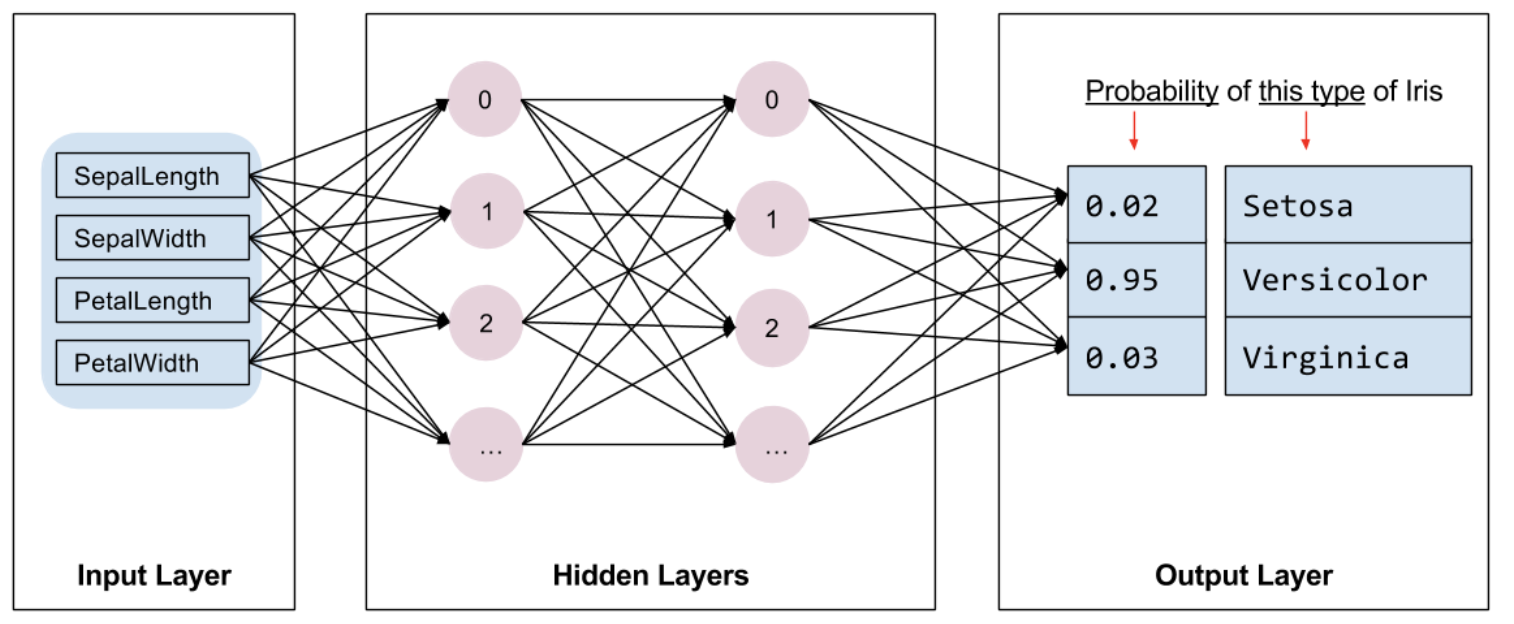下面是评估器(Estimator)编程的基本概念：
Estimator是TensorFlow对完整模型的高级表示。它处理初始化，记录，保存和恢复以及许多其他功能的细节。
Estimator是从tf.estimator.Estimator派生的通用类。 TensorFlow提供了一系列定制的评估器（例如LinearRegressor）来实现常用的ML算法。除此之外，也可以编写自己的定制评估器。但建议在刚开始使用TensorFlow时使用内置的Estimator。在获得内置的Estimator的专业知识后，就可以通过创建定制Estimator来优化模型。

要根据内置的Estimator编写TensorFlow程序，必须执行以下任务：

• 创建一个或多个输入函数。
• 定义模型的特征列。
• 实例化Estimator，指定特征列和各种超参数。
• 在Estimator对象上调用一个或多个方法，传递适当的输入函数作为数据源。

2. lstm预测正弦函数

下面是利用lstm来实现预测正弦函数sin。

#!/usr/bin/env python3
# -*- coding: utf-8 -*-

import numpy as np
import tensorflow as tf
from tensorflow.contrib.learn.python.learn.estimators.estimator import SKCompat
from tensorflow.python.ops import array_ops as array_ops_

import matplotlib.pyplot as plt

learn = tf.contrib.learn

# 设置神经网络的参数
HIDDEN_SIZE = 30 # LSTM中隐藏的节点个数
NUM_LAYERS = 2  # LSTM的层数

TIMESTEPS = 10  # 循环神经网路的截断长度
TRAINING_STEPS = 3000  # 循环轮数
BATCH_SIZE = 32 # batch大小

TRAINING_EXAMPLES = 10000  # 训练数据的个数
TESTING_EXAMPLES = 1000    # 测试数据的个数
SAMPLE_GAP = 0.01          # 采样间隔

# 定义生成正弦数据的函数
def generate_data(seq):
X = []
y = []
# 序列的第i项和后面的TIMESTEPS-1项合在一起作为输入；第i + TIMESTEPS项作为输
# 出。即用sin函数前面的TIMESTEPS个点的信息，预测第i + TIMESTEPS个点的函数值。
for i in range(len(seq) - TIMESTEPS):
X.append([seq[i: i + TIMESTEPS]])
y.append([seq[i + TIMESTEPS]])
return np.array(X, dtype=np.float32), np.array(y, dtype=np.float32)

# 用正弦函数生成训练和测试数据集合。
test_start = (TRAINING_EXAMPLES + TIMESTEPS) * SAMPLE_GAP   # (10000+10)*0.01=100
test_end = test_start + (TESTING_EXAMPLES + TIMESTEPS) * SAMPLE_GAP # 100+(1000+10)*0.01=110
train_X, train_y = generate_data(np.sin(np.linspace(
0, test_start, TRAINING_EXAMPLES + TIMESTEPS, dtype=np.float32)))
test_X, test_y = generate_data(np.sin(np.linspace(
test_start, test_end, TESTING_EXAMPLES + TIMESTEPS, dtype=np.float32)))

#  定义lstm模型
def lstm_model(X, y, is_training):
# 使用多层的LSTM结构。
cell = tf.nn.rnn_cell.MultiRNNCell([
tf.nn.rnn_cell.BasicLSTMCell(HIDDEN_SIZE)
for _ in range(NUM_LAYERS)])

# 使用TensorFlow接口将多层的LSTM结构连接成RNN网络并计算其前向传播结果。
outputs, _ = tf.nn.dynamic_rnn(cell, X, dtype=tf.float32)
#print(len(outputs))
output = outputs[:, -1, :]

# 对LSTM网络的输出再做加一层全链接层并计算损失。注意这里默认的损失为平均平方差损失函数。
predictions = tf.contrib.layers.fully_connected(
output, 1, activation_fn=None)

# 只在训练时计算损失函数和优化步骤。测试时直接返回预测结果。
if not is_training:
return predictions, None, None

# 计算损失函数。
loss = tf.losses.mean_squared_error(labels=y, predictions=predictions)

# 创建模型优化器并得到优化步骤。
train_op = tf.contrib.layers.optimize_loss(
loss, tf.train.get_global_step(),
return predictions, loss, train_op

"""
定义测试方法
"""
def run_eval(sess, test_X, test_y):
# 将测试数据以数据集的方式提供给计算图。
ds = tf.data.Dataset.from_tensor_slices((test_X, test_y))
ds = ds.batch(1)
X, y = ds.make_one_shot_iterator().get_next()

# 调用模型得到计算结果。这里不需要输入真实的y值。
with tf.variable_scope("model", reuse=True):
prediction, _, _ = lstm_model(X, [0.0], False)

# 将预测结果存入一个数组。
predictions = []
labels = []
for i in range(TESTING_EXAMPLES):
p, l = sess.run([prediction, y])
predictions.append(p)
labels.append(l)

# 计算mse作为评价指标。
predictions = np.array(predictions).squeeze()
labels = np.array(labels).squeeze()
rmse = np.sqrt(((predictions - labels) ** 2).mean(axis=0))
print("Root Mean Square Error is: %f" % rmse)

#对预测的sin函数曲线进行绘图。
plt.figure()
plt.plot(predictions, label='predictions')
plt.plot(labels, label='real_sin')
plt.legend()
plt.show()

"""
执行训练和测试
"""
# 将训练数据以数据集的方式提供给计算图。
print(train_X.shape)
print(train_y.shape)
ds = tf.data.Dataset.from_tensor_slices((train_X, train_y))
ds = ds.repeat().shuffle(1000).batch(BATCH_SIZE)

X, y = ds.make_one_shot_iterator().get_next()

# 定义模型，得到预测结果、损失函数，和训练操作。
with tf.variable_scope("model"):
_, loss, train_op = lstm_model(X, y, True)

with tf.Session() as sess:
sess.run(tf.global_variables_initializer())
# 测试在训练之前的模型效果。
print ("Evaluate model before training.")
run_eval(sess, test_X, test_y)

# 训练模型。
for i in range(TRAINING_STEPS):
_, l = sess.run([train_op, loss])
if i % 1000 == 0:
print("train step: " + str(i) + ", loss: " + str(l))

# 使用训练好的模型对测试数据进行预测。
print ("Evaluate model after training.")
run_eval(sess, test_X, test_y)

运行结果：

(10000, 1, 10)
(10000, 1)
Evaluate model before training.
Root Mean Square Error is: 0.688176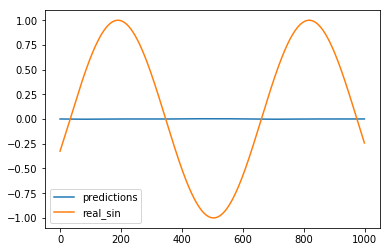train step: 0, loss: 0.5273397
train step: 1000, loss: 0.000585775
train step: 2000, loss: 0.0003093369
Evaluate model after training.
Root Mean Square Error is: 0.007838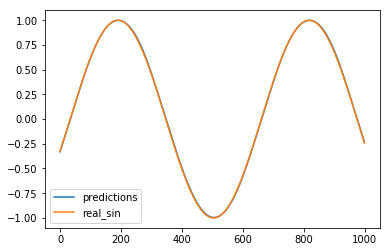预测曲线基本重合与目标曲线。

3. tf.data.Dataset.from_tensor_slices和repeat()、shuffle()、batch()使用

1. tf.data.Dataset.from_tensor_slices使用

（1）简单数组数列使用

import tensorflow as tf
import numpy as np
## 数据集对象实例化
dataset = tf.data.Dataset.from_tensor_slices(np.array([1.0, 2.0, 3.0, 4.0, 5.0]))
# # 迭代器对象实例化
iterator = dataset.make_one_shot_iterator()
one_element = iterator.get_next()
with tf.Session() as sess:
try:
while True:
print(sess.run(one_element))
except tf.errors.OutOfRangeError:
print("end!")

运行结果：

1.0
2.0
3.0
4.0
5.0
end!

（2）多维数据使用

import tensorflow as tf
import numpy as np

dataset = tf.data.Dataset.from_tensor_slices(np.random.uniform(size=(5, 2)))
print('dataset:',dataset)
iterator = dataset.make_one_shot_iterator()
one_element = iterator.get_next()
with tf.Session() as sess:
try:
while True:
print(sess.run(one_element))
except tf.errors.OutOfRangeError:
print("end!")

运行结果：

dataset: <TensorSliceDataset shapes: (2,), types: tf.float64>
[0.1792582 0.9751422]
[0.19793807 0.33374795]
[0.03978658 0.6808261 ]
[0.98788989 0.07403864]
[0.32259662 0.50171158]
end!

传入的数值是一个矩阵，它的形状为(5, 2)，tf.data.Dataset.from_tensor_slices就会切分它形状上的第一个维度，最后生成的dataset中一个含有5个元素(但这里的元素个数不知道)，每个元素的形状是(2, )，即每个元素是矩阵的一行。

（3）字典使用

import tensorflow as tf
import numpy as np

# 数据集对象实例化
dataset = tf.data.Dataset.from_tensor_slices(
{
"a": np.array([1.0, 2.0, 3.0, 4.0, 5.0]),
"b": np.random.uniform(size=(5, 2))
})

# 迭代器对象实例化
iterator = dataset.make_one_shot_iterator()
one_element = iterator.get_next()

with tf.Session() as sess:
try:
while True:
print(sess.run(one_element))
except tf.errors.OutOfRangeError:
print("end!")

运行结果：

{'a': 1.0, 'b': array([0.04879087, 0.22908515])}
{'a': 2.0, 'b': array([0.11519196, 0.78684246])}
{'a': 3.0, 'b': array([0.86009348, 0.41073689])}
{'a': 4.0, 'b': array([0.80116343, 0.4113549 ])}
{'a': 5.0, 'b': array([0.545203  , 0.23978585])}
end!

对于复杂的情形，比如元素是一个python中的元组或者字典：在图像识别中一个元素可以是｛”image”:image_tensor,”label”:label_tensor｝的形式。 如上所示，这时函数会分别切分”label”中的数值以及”fea”中的数值，最后总dataset中的一个元素就是类似于{ “a”:1.0, “b”:[0.9,0.1] }的形式。

（4）复杂的tuple组合数据

import tensorflow as tf
import numpy as np

dataset = tf.data.Dataset.from_tensor_slices(
(np.array([1.0, 2.0, 3.0, 4.0, 5.0]), np.random.uniform(size=(5, 2)))
)

iterator = dataset.make_one_shot_iterator()
one_element = iterator.get_next()
with tf.Session() as sess:
try:
while True:
print(sess.run(one_element))
except tf.errors.OutOfRangeError:
print("end!")

运行结果：

(1.0, array([0.61708973, 0.73534924]))
(2.0, array([0.64866959, 0.04011806]))
(3.0, array([0.07455173, 0.02324372]))
(4.0, array([0.53580828, 0.19761375]))
(5.0, array([0.63886913, 0.56859266]))
end!

1. repeat()、shuffle()、batch()使用
Dataset支持一类特殊的操作：Transformation。一个Dataset通过Transformation变成一个新的Dataset。通常我们可以通过Transformation完成数据变换，打乱，组成batch，生成epoch等一系列操作。

（1）batch的使用

import tensorflow as tf
import numpy as np

# 数据集对象实例化
dataset = tf.data.Dataset.from_tensor_slices(
{
"a": np.array([1.0, 2.0, 3.0, 4.0, 5.0]),
"b": np.random.uniform(size=(5, 2))
})

dataset = dataset.batch(2)

# 迭代器对象实例化
iterator = dataset.make_one_shot_iterator()
one_element = iterator.get_next()

with tf.Session() as sess:
try:
while True:
print(sess.run(one_element))
except tf.errors.OutOfRangeError:
print("end!")

运行结果：

{'a': array([1., 2.]), 'b': array([[0.34187133, 0.40294537],
[0.14411398, 0.06565229]])}
{'a': array([3., 4.]), 'b': array([[0.58521367, 0.1550559 ],
[0.39541026, 0.87366261]])}
{'a': array([5.]), 'b': array([[0.10484032, 0.75835755]])}
end!

可以看出每两个数据组成一个batch
（2）shuffle的使用
shuffle的功能为打乱dataset中的元素，它有一个参数buffersize，表示打乱时使用的buffer的大小，建议设置不要太小，一般是1000：

import tensorflow as tf
import numpy as np

dataset = tf.data.Dataset.from_tensor_slices(
{
"a": np.array([1.0, 2.0, 3.0, 4.0, 5.0]),
"b": np.random.uniform(size=(5, 2))
})

dataset = dataset.shuffle(buffer_size=1000)

iterator = dataset.make_one_shot_iterator()
one_element = iterator.get_next()
with tf.Session() as sess:
try:
while True:
print(sess.run(one_element))
except tf.errors.OutOfRangeError:
print("end!")

运行结果：

{'a': 3.0, 'b': array([0.60925085, 0.60532416])}
{'a': 2.0, 'b': array([0.66688525, 0.29313125])}
{'a': 1.0, 'b': array([0.10190033, 0.3020232 ])}
{'a': 4.0, 'b': array([0.20525341, 0.50205214])}
{'a': 5.0, 'b': array([0.4151064 , 0.00696569])}

（3）repeat的使用

repeat的功能就是将整个序列重复多次，主要用来处理机器学习中的epoch，假设原先的数据是一个epoch，使用repeat(2)就可以将之变成2个epoch：

import tensorflow as tf
import numpy as np

dataset = tf.data.Dataset.from_tensor_slices(
{
"a": np.array([1.0, 2.0, 3.0, 4.0, 5.0]),
"b": np.random.uniform(size=(5, 2))
})

dataset = dataset.repeat(3)

iterator = dataset.make_one_shot_iterator()
one_element = iterator.get_next()
with tf.Session() as sess:
try:
while True:
print(sess.run(one_element))
except tf.errors.OutOfRangeError:
print("end!")

运行结果：

{'a': 1.0, 'b': array([0.14897444, 0.444479  ])}
{'a': 2.0, 'b': array([0.10113141, 0.89834262])}
{'a': 3.0, 'b': array([0.18748515, 0.95026317])}
{'a': 4.0, 'b': array([0.56672754, 0.26056881])}
{'a': 5.0, 'b': array([0.5487119 , 0.28498332])}
{'a': 1.0, 'b': array([0.14897444, 0.444479  ])}
{'a': 2.0, 'b': array([0.10113141, 0.89834262])}
{'a': 3.0, 'b': array([0.18748515, 0.95026317])}
{'a': 4.0, 'b': array([0.56672754, 0.26056881])}
{'a': 5.0, 'b': array([0.5487119 , 0.28498332])}
{'a': 1.0, 'b': array([0.14897444, 0.444479  ])}
{'a': 2.0, 'b': array([0.10113141, 0.89834262])}
{'a': 3.0, 'b': array([0.18748515, 0.95026317])}
{'a': 4.0, 'b': array([0.56672754, 0.26056881])}
{'a': 5.0, 'b': array([0.5487119 , 0.28498332])}
end!

展开全文TFlearn iris分类 lstm预测正弦函数
• 本文采用一个较为简单的例子，来介绍循环神经网络。 文中的代码按顺序复制下来可以运行，并得出结果。 关于循环神经网络的原理，后续会专门再介绍，详细关注我的博客。对于大多数程序员来说，通过程序来理解，可能...

本文采用一个较为简单的例子，来介绍循环神经网络。 文中的代码按顺序复制下来可以运行，并得出结果。

关于循环神经网络的原理，后续会专门再介绍，详细关注我的博客。对于大多数程序员来说，通过程序来理解，可能更为直观。

例子： 输入3行4列的矩阵数据，如

[1,  2,  5,  6],
[5,  7,  7,  8],
[3,  4,  5,  7]

输出：

[1,   3,   7,  11],
[5, 12, 14,  15],
[3,   7,   9,  12]

分析规律： 输出第1列为输入的第1列， 输出第2列为输入的第1列和第2列之和， 输出的第3列为输入的第2列和第3列之和，依次类推。那么如何通过神经网络来训练这个模型呢？ 可以看到里面有LSTM（长短文本分析）的特征，所以，我们很自然的想到用循环神经网络来训练。因为数据量不多，这个地方用一层网络就可以了。 下面请看代码

首先导入依赖包：

import tensorflow as tf
from tensorflow.contrib import rnn
tf.reset_default_graph()

创建一个模型类， 这个类中包含RNN训练参数初始化，损失函数及优化器的构建，模型生成方法，模型训练方法，以及测试方法

class SeriesPredictor:
def __init__(self, input_dim, seq_size, hidden_dim=10):
# 网络参数
self.input_dim = input_dim  # 输入维度
self.seq_size = seq_size # 时序长度
self.hidden_dim = hidden_dim  # 隐藏层维度

# 权重参数W与输入X及标签Y
self.W_out = tf.Variable(tf.random_normal([hidden_dim, 1]), name="W_out")
self.b_out = tf.Variable(tf.random_normal(), name='b_out')
self.x = tf.placeholder(tf.float32, [None, seq_size, input_dim])
self.y = tf.placeholder(tf.float32, [None, seq_size])

# 均方误差求损失值，并使用梯度下降
self.cost = tf.reduce_mean(tf.square(self.model() - self.y))

self.saver = tf.train.Saver()

def model(self):
'''
:param x: inpouts of size [T, batch_size, input_size]
:param W: matrix of fully-connected output layer weights
:param b: vector of fully-connected output layer biases
'''
# BasicLSTMCell基本的RNN类， 建立hidden_dim个CELL
cell = rnn.BasicLSTMCell(self.hidden_dim)
# dynamic_rnn 动态RNN， cell生成好的cell类对象， self.x是一个张量， 一般是三维张量[Batch_size, max_time(序列时间X0-Xt), X具体输入]
outputs, states = tf.nn.dynamic_rnn(cell, self.x, dtype=tf.float32)  # (?, seq_size, hidden_dim)
num_examples = tf.shape(self.x)
tf_expand = tf.expand_dims(self.W_out, 0)
tf_tile = tf.tile(tf_expand, [num_examples, 1, 1])  # 将第一维扩大为num_examples维  (?, hidden_dim, 1)
out = tf.matmul(outputs, tf_tile) + self.b_out  # (?, seq_size, 1)
print(out)
out = tf.squeeze(out)
return out

def train(self, train_x, train_y):
with tf.Session() as sess:
tf.get_variable_scope().reuse_variables()  # 变量可重复利用
sess.run(tf.global_variables_initializer())
for i in range(1000):
_, mse = sess.run([self.train_op, self.cost], feed_dict={self.x: train_x, self.y: train_y})
if i % 100 == 0:
print(i, mse)
save_path = self.saver.save(sess, './model')
print('Model saved to {}'.format(save_path))

def test(self, test_x):
with tf.Session() as sess:
tf.get_variable_scope().reuse_variables()
self.saver.restore(sess, './model')
output = sess.run(self.model(), feed_dict={self.x: test_x})
return output

最后写一个main函数，用训练数据训练网络，并用测试数据测试

if __name__ == '__main__':
predictor = SeriesPredictor(input_dim=1, seq_size=4, hidden_dim=10)
train_x = [[, , , ],
[, , , ],
[, , , ]]

train_y = [[1, 3, 7, 11],
[5, 12, 14, 15],
[3, 7, 9, 12]]

predictor.train(train_x, train_y)

test_x = [[, , , ],
[, , , ]]
test_y = [[, , , ],
[, , , ]]
pred_y = predictor.test(test_x)

print("\n开始测试!\n")

for i, x in enumerate(test_x):
print("当前输入{}".format(x))
print("应该输出{}".format(test_y[i]))
print("训练模型的输出{}".format(pred_y[i]))

查看运行结果：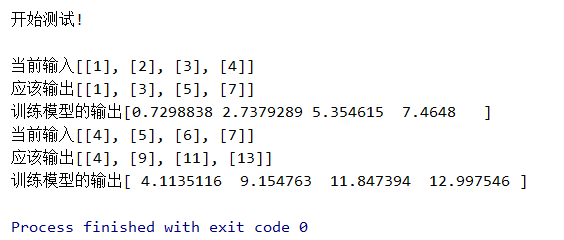可以看到模型预测的结果与应该输入的结果是比较接近的。 在实际运用中，经常会调整一些参数，在不导致过拟合和欠拟合的条件下，使得网络模型更精确，比如加减神经元、调整网络层数、调整训练次数等。

展开全文深度学习 tensorflow 神经网络 自然语言处理 机器学习
• RNN循环神经网络实例

千次阅读 2019-04-22 21:56:20
最近又忍不住把RNN这一块儿的东西...关于RNN循环神经网络的具体概念我就不细讲了，稍微把概念给提下吧，然后会说说其变形模型，以及会给出两个demo讲述其在不同领域的应用，方便大家理解！ 1. RNN循环神经网络介绍 ...

最近又忍不住把RNN这一块儿的东西给过了一遍，感觉还是有一些收获！所以想着给记录下来，因为也看到有人给我提意见说：我写的关于算法的文章太多了，还是要好好搞学术研究，所以就想着最近多更几篇关于深度学习网络方面的文章。

关于RNN循环神经网络的具体概念我就不细讲了，稍微把概念给提下吧，然后会说说其变形模型，以及会给出两个demo讲述其在不同领域的应用，方便大家理解！

1. RNN循环神经网络介绍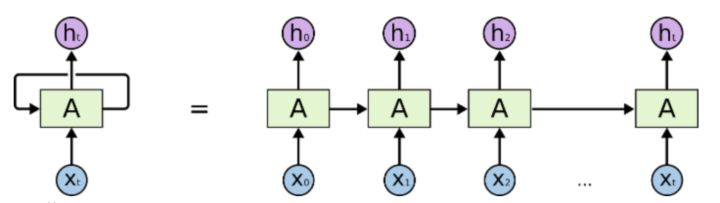上面这张图算是最最最经典的也是最一般的RNN网络结构了。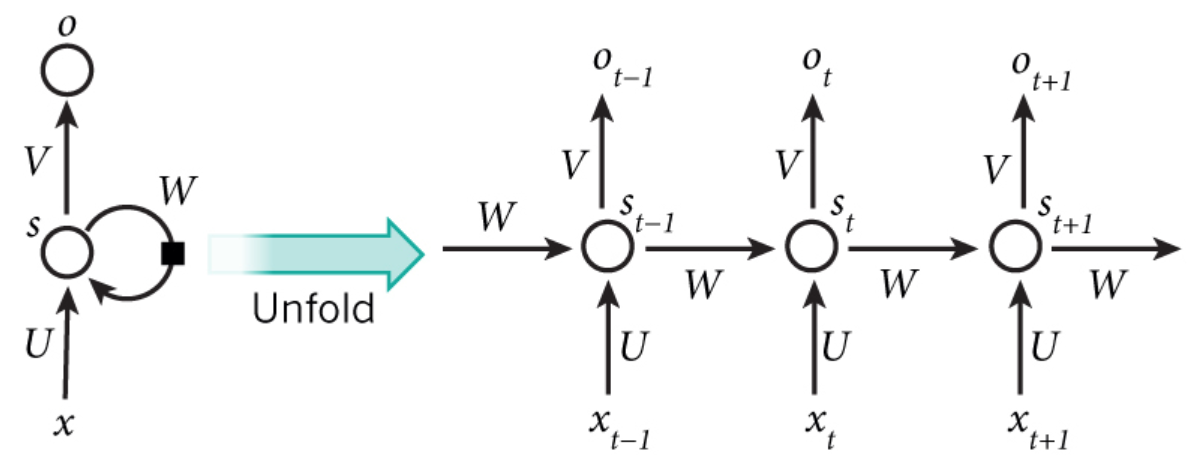因为要讲解步骤，所以用这张图来表示步骤，上图其实是把RNN给展开成我们熟悉的全连接神经网络了，这样我们会比较熟悉。步骤如下：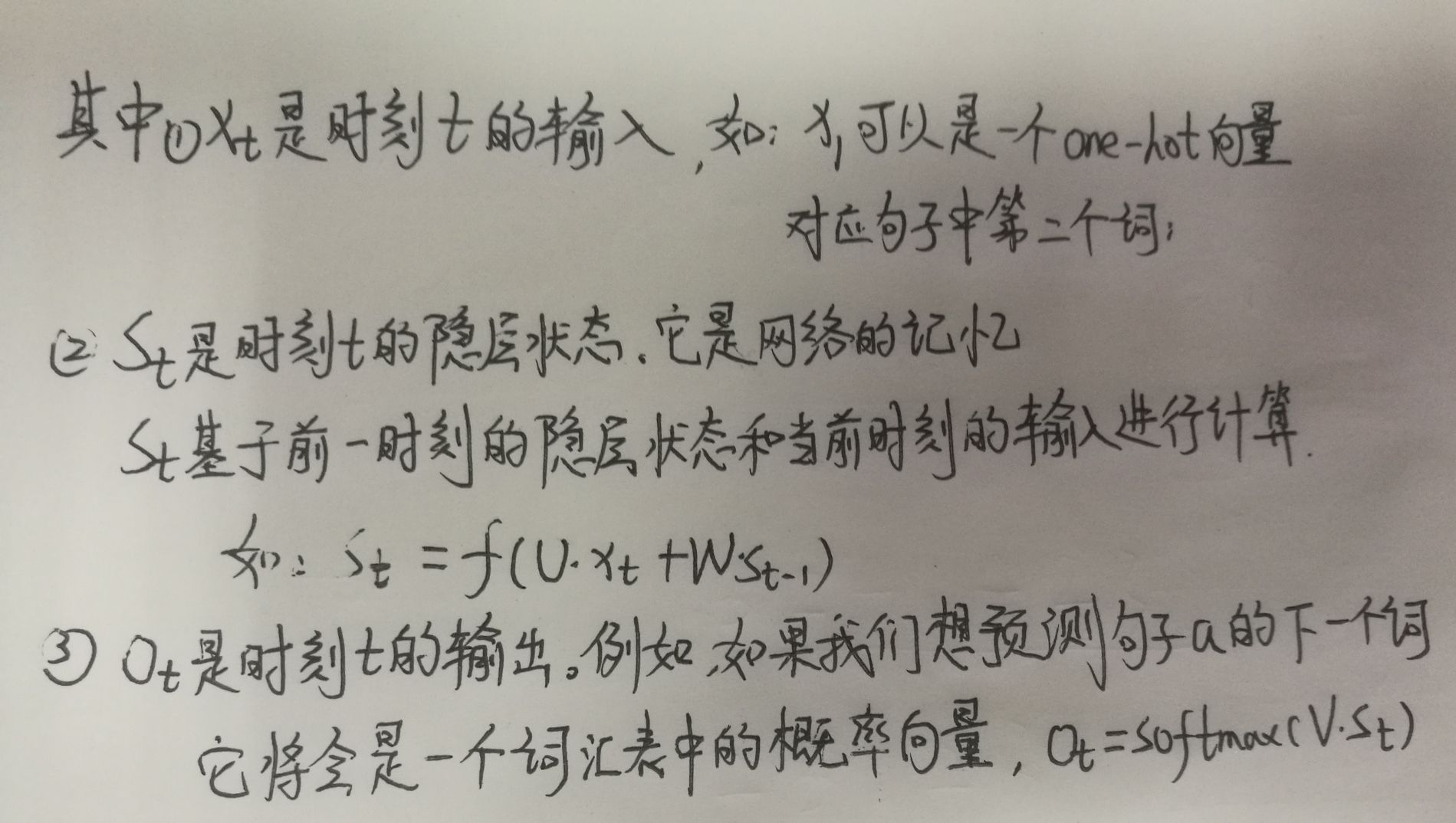基于上述步骤可实现一简单的RNN神经网络，核心流程如下demo所示：

import numpy as np
import os
os.environ["TF_CPP_MIN_LOG_LEVEL"] = "2"

X = [1, 2]
state = [0.0, 0.0]
# 定义不同输入部分的权重
w_cell_state = np.asarray([[0.1, 0.2], [0.3, 0.4]])
w_cell_input = np.asarray([0.5, 0.6])
b_cell = np.asarray([0.1, -0.1])
# 定义输出层的权重
w_output = np.asarray([[0.1], [0.2]])
b_output = 0.1
# 按照时间顺序执行循环神经网络的前向传播过程
for i in range(len(X)):
before_activation = np.dot(state, w_cell_state) + X[i]*w_cell_input+b_cell
state = np.tanh(before_activation)
# 计算当前时刻的最终输出
final_output = np.dot(state, w_output) + b_output
# 输出每一时刻的信息
print("before_activation", before_activation)
print("state", state)
print("final_output", final_output)

只说这个最简单的网络，肯定是没有意思的！所以接下来我会分享一个用RNN来识别手写字体的demo。

2. RNN神经网络识别手写字体

import tensorflow as tf
from tensorflow.examples.tutorials.mnist import input_data
from tensorflow.contrib import rnn
import os
os.environ["TF_CPP_MIN_LOG_LEVEL"] = "2"

# 载入数据

# 设置模型的参数
# 对应输入图片的宽
n_inputs = 28
# 对应输入图片的行
max_time = 28
# 隐藏单元，实质上不是神经元，即LSTM单元
lstm_size = 100
# 图片总共有10个分类
n_classes = 10
# 每批次总共有50个样本
batch_size = 50
n_batch = mnist.train.num_examples//batch_size

# 这里的None=50
x = tf.placeholder(tf.float32, [None, 784])
y = tf.placeholder(tf.float32, [None, 10])

# 初始化权值
weights = tf.Variable(tf.truncated_normal([lstm_size, n_classes], stddev=0.1))
# 初始化偏置值
biases = tf.Variable(tf.constant(0.1, shape=[n_classes]))

# 定义RNN网络
def RNN(X, weights, biases):
inputs = tf.reshape(X, [-1, max_time, n_inputs])
lstm_cell = rnn.BasicLSTMCell(lstm_size)
# outputs维度为shape=(?, 28, 100)；final_state维度为shape=(?, 100)
outputs, final_state = tf.nn.dynamic_rnn(lstm_cell, inputs, dtype=tf.float32)
print("shape(outputs)", outputs)
print("shape(final_state)", final_state)
# 如果使用Seq2Seq模型，则调用outputs；反之则使用final_state
results = tf.nn.softmax(tf.matmul(final_state, weights)+biases)
return results

# 计算RNN的返回结果
prediction = RNN(x, weights, biases)
# 损失函数--交叉熵
cross_entropy = tf.reduce_mean(tf.nn.softmax_cross_entropy_with_logits_v2(labels=y, logits=prediction))
# 结果放在一个布尔型列表中
crroect_prediction = tf.equal(tf.argmax(y, 1), tf.argmax(prediction, 1))
# 求准确率
accuracy = tf.reduce_mean(tf.cast(crroect_prediction, tf.float32))

# 开始训练模型
with tf.Session() as sess:
sess.run(tf.global_variables_initializer())
for epoch in range(10):
for batch in range(n_batch):
batch_xs, batch_ys = mnist.train.next_batch(batch_size)
sess.run(train_op, feed_dict={x: batch_xs, y:batch_ys})
acc = sess.run(accuracy, feed_dict={x: mnist.test.images, y: mnist.test.labels})
print("Iter "+str(epoch)+", Testing Accuracy="+str(acc))

这个demo里有处地方我需要强调一下：

outputs, final_state = tf.nn.dynamic_rnn(lstm_cell, inputs, dtype=tf.float32)
# 如果使用Seq2Seq模型，则调用outputs；反之则使用final_state
results = tf.nn.softmax(tf.matmul(final_state, weights)+biases)

这里面outputs维度为shape=(?, 28, 100)；final_state维度为shape=(?, 100)，具体结构如下图所示：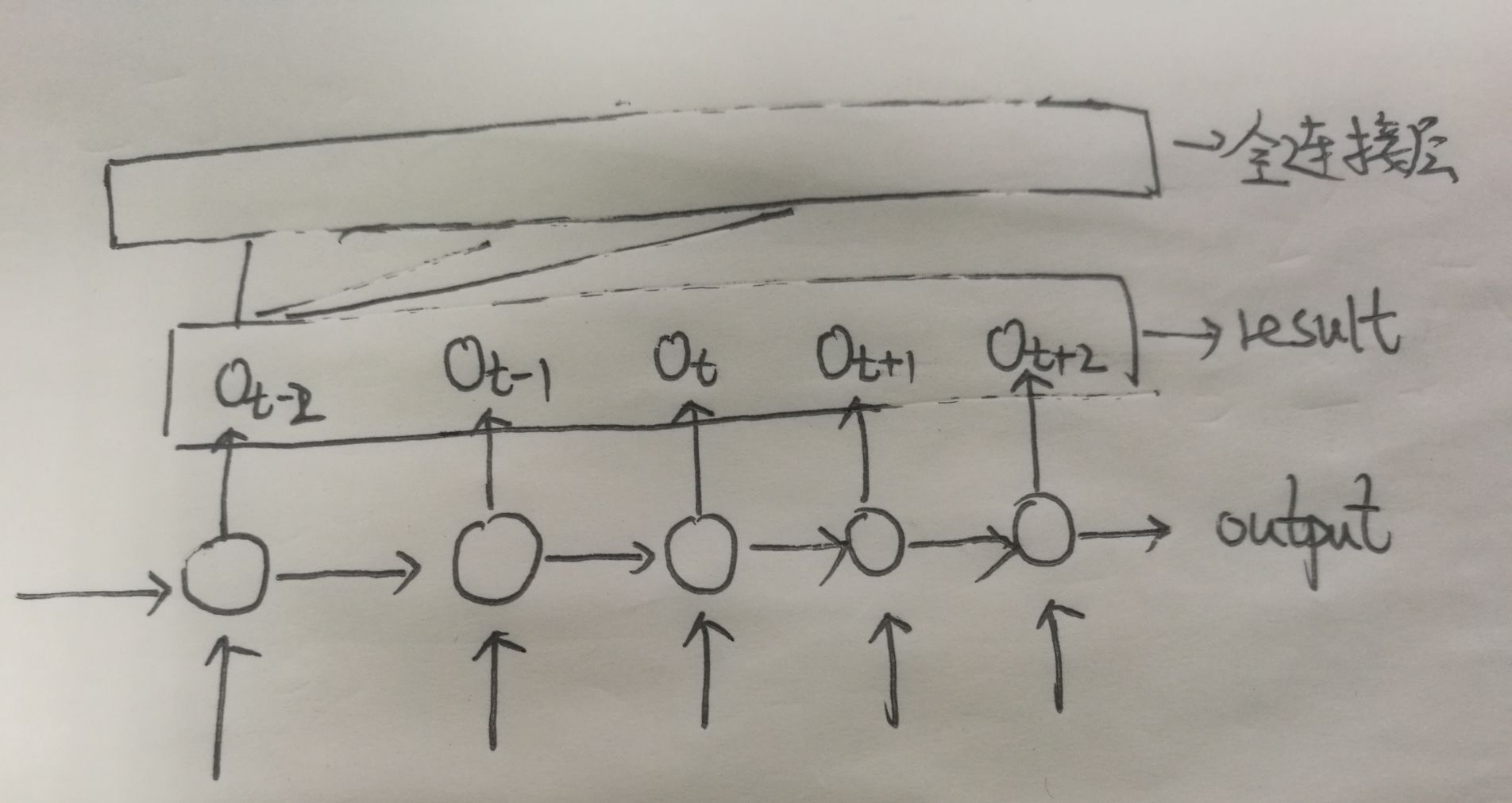在这个demo里我们是没用到output这个LSTM cell的，不是说它没用哈，而是在后面提到的Seq2Seq模型中会用到这个值。

3. RNN神经网络预测下一个字符

不知道大家发现没有，上面讲到的这个最基础的RNN模型中输入数据的“维度”与输出数据的“维度”是一一对应的，即Xt与Ot是相对应的。但是在实际应用中这种情形太少了，跟多的是N->M的关系，即输入数据的维度”与输出数据的“维度”是不同的。比如在机器翻译应用中，将一段汉语翻译成英文。汉语是：我英语不好，翻译成英文是：my English is poor 此处N=5,M=4明显是N与M不相等的情形，此时我们要怎么解决这个模型呢？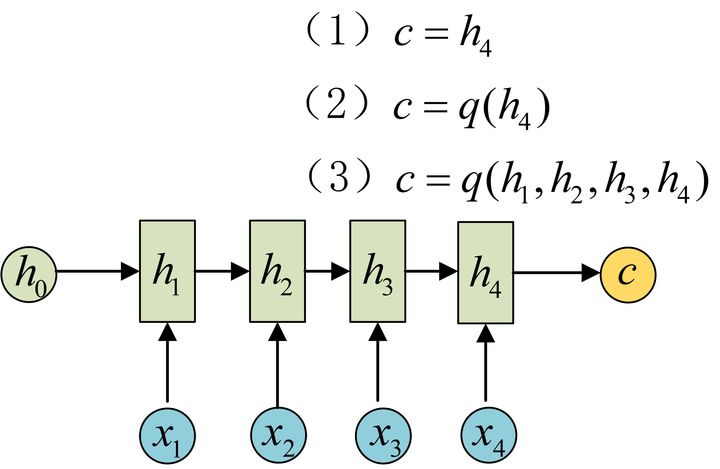那问题就来了，此时我们要怎么解决这个模型呢？

为此得引入一种新的模型，这种结构又叫Encoder-Decoder模型，也可以称之为Seq2Seq模型。如下图所示：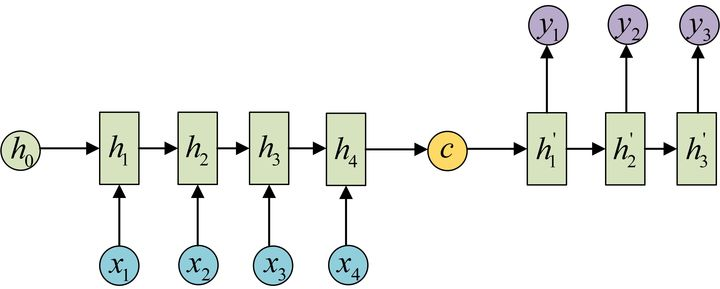还有一种做法是将c当做每一步的输入：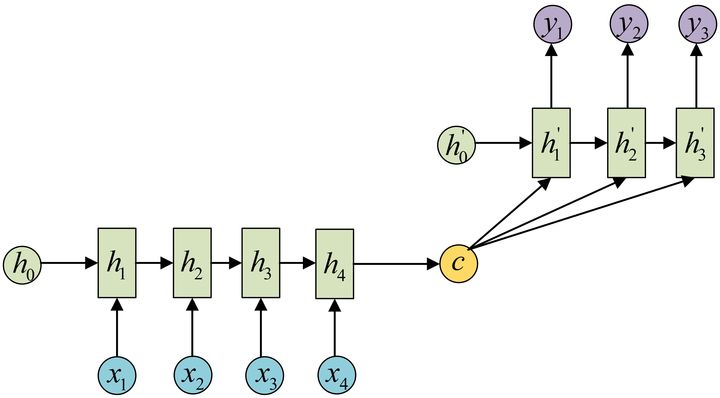按照这个模型，我接下来将会给出能预测下一个字符的RNN模型

import tensorflow as tf
from tensorflow import contrib
import numpy as np
import os
os.environ["TF_CPP_MIN_LOG_LEVEL"] = "2"

sample = "这是一个基于tensorflow的RNN短句子练习"
# 去重放入列表中
idx2char = list(set(sample))
print("idx2char", idx2char)
# 转换为字典，其中把字母作键，索引作为值
char2idx = {c: i for i, c in enumerate(idx2char)}
# 在字典里取出对应值，因为在idx2char中原sample句子的顺序已经被打乱
sample_idx = [char2idx[c] for c in sample]
x_data = [sample_idx[:-1]]
y_data = [sample_idx[1:]]

# 设置该模型的一些参数
dic_size = len(char2idx)
rnn_hidden_size = len(char2idx)
num_classes = len(char2idx)
batch_size = 1
sequence_length = len(sample) - 1

X = tf.placeholder(tf.int32, [None, sequence_length])
Y = tf.placeholder(tf.int32, [None, sequence_length])
# 将input转化为one-hot类型数据输出，此时X的维度变为[None, sequence_length, num_classes]
X_one_hot = tf.one_hot(X, num_classes)

cell = tf.contrib.rnn.BasicLSTMCell(num_units=rnn_hidden_size, state_is_tuple=True)
initial_state = cell.zero_state(batch_size, tf.float32)
outputs, states = tf.nn.dynamic_rnn(cell, X_one_hot, initial_state=initial_state, dtype=tf.float32)
# 加一层全连接层，相当于加一层深度，使预测更准确
outputs = contrib.layers.fully_connected(inputs=outputs, num_outputs=num_classes, activation_fn=None)
print("outputs", tf.shape(outputs))
weights = tf.ones([batch_size, sequence_length])
# 此处包装了encoder和decoder
sequence_loss = tf.contrib.seq2seq.sequence_loss(logits=outputs, targets=Y, weights=weights)
loss = tf.reduce_mean(sequence_loss)

prediction = tf.argmax(outputs, axis=2)

with tf.Session() as sess:
sess.run(tf.global_variables_initializer())
for i in range(3000):
l, _ = sess.run([loss, train], feed_dict={X: x_data, Y: y_data})
result = sess.run(prediction, feed_dict={X: x_data})
# print char using dic
result_str = [idx2char[c] for c in np.squeeze(result)]
print(i, "loss:", l, "Prediction:", "".join(result_str))
print(len(result_str))

模型Run起来的结果如下：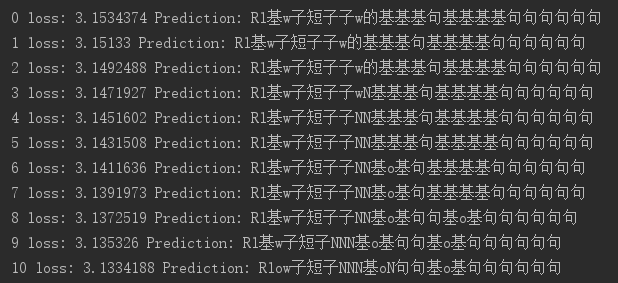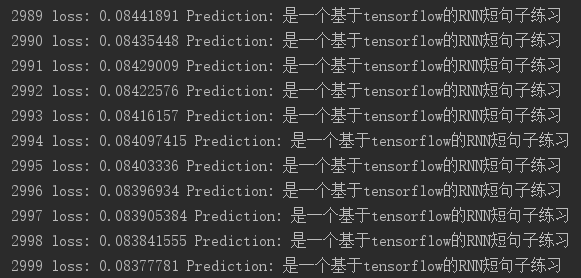可以看到：效果还是不错的！暂时就分享到这儿了，有想到更好的东西再来补充！

展开全文python RNN循环神经网络
• 循环神经网络(Recurrent Neural Network, RNN)是用来建模序列化数据的一种主流深度学习模型。RNN将神经元串行起来，每个神经元能用它的内部变量保存之间输入的序列信息来把整个序列浓缩成抽象表示，并据此进行分类或...人工智能 深度学习 神经网络
•  接着上文的CNN来说，CNN通常用于计算机视觉领域那么这个循环神经网络（RNN）是什么的干活？相比于计算机视觉RNN通常作用于语言处理，目前最为常见的自然语言处理就是通过RNN的‘同胞’实现的。或许你会疑问既然CNN...深度学习
• 本文综合整理常用的神经网络，包括生物神经网络、人工神经网络、卷积神经网络、循环神经网络、生成对抗网络；参考了许多高校的课程、论文、博客和视频等。文章的结构是先进行概念了解，然后结合图片、结构图、一步...神经网络 人工神经网络 人工智能 卷积神经网络 深度学习
• 循环神经网络（原理+代码）

千次阅读 多人点赞 2019-07-06 18:09:09
在传统的全连接神经网络中，从输入层到隐藏层再到输出层，每一层之间的节点是无连接的，因为输入和输出是独立的，所以这种普通的神经网络对于序列数据的处理是无能为力的。而现实中，绝大多数的数据都是序列数据...RNN 情感分析
• 文章目录1. 什么是RNN1.1 RNN的应用1.2 为什么有了CNN，还要RNN?1.3 RNN的网络结构1.4 双向RNN1.5 BPTT算法2....循环神经网络（Recurrent Neural Network, RNN）是一类以序列（sequence）数据为输入，在序...RNN 循环神经网络 BPTT 深度学习 机器学习
• 浅谈循环神经网络（RNN）

千次阅读 2018-05-22 15:49:08
循环神经网络应用场景比较多，比如暂时能写论文，写程序，写诗，但是，（总是会有但是的），但是他们现在还不能正常使用，学习出来的东西没有逻辑，所以要想真正让它更有用，路还很远。这是一般的神经网络应该有的...
• 通过keras例子理解LSTM 循环神经网络(RNN)

万次阅读 多人点赞 2018-05-29 11:05:54
一个强大而流行的循环神经网络(RNN)的变种是长短期模型网络(LSTM)。 它使用广泛，因为它的架构克服了困扰着所有周期性的神经网络梯度消失和梯度爆炸的问题，允许创建非常大的、非常深的网络。 与其他周期性的神...RNN LSTM keras
• 1、RNN的简单介绍        我们前面提到的无论是人工神经网络还是CNN卷积神经网络，他们的前提都是：元素之间是相互独立的，...因此，我们就需要使用循环神经网络了，他的本质是：像算法 人工智能 神经网络
• 使用循环神经网络预测温度

千次阅读 2020-12-31 21:42:03
keras每个循环层都有两个与dropout相关的参数： dropout: 浮点数，指定该层输入单元的dropout比率 recurrent_dropout: 指定循环单元的dropout比率 注意，添加dropout后，网络需要更长的时间才能完全收敛，所以网络...rnn gru
• 深度学习之RNN(循环神经网络)

万次阅读 多人点赞 2018-05-28 20:49:09
一 RNN概述  前面我们叙述了BP算法, CNN算法, 那么为什么还会有RNN呢?... 细想BP算法,CNN(卷积神经网络)我们会发现, 他们的输出都是只考虑前一个输入的影响而不考虑其它时刻输入的影响, 比如简单的猫,狗,手写数...RNN 循环神经网络 深度学习
• 双向循环神经网络(BiRNN)

千次阅读 2020-09-19 09:31:39
双向循环神经网络（BRNN）的基本思想是提出每一个训练序列向前和向后分别是两个循环神经网络（RNN），而且这两个都连接着一个输出层。这个结构提供给输出层输入序列中每一个点的完整的过去和未来的上下文信息。下图...
•    这篇文章很多内容是参考：...  循环神经网络(Recurrent Neural Networks，RNNs)已经在众多自然语言处理(Natural Langu...自然语言处理 神经网络
• 循环神经网络简介 循环神经网络（recurrent neural network, RNN）源自1982年由Saratha Sathasivam提出的霍普菲尔德网络。霍普菲尔德网络因为实现困难，在提出时并且没有被合适地应用。该网络结构也于1986年后被全...RNN 循环神经网络
• 将神经网络模型训练好之后，在输入层给定一个x，通过网络之后就能够在输出层得到特定的y，那么既然有了这么强大的模型，为什么还需要RNN（循环神经网络）呢？ 1 为什么需要RNN（循环神经网络） 他们都只能单独的取...rnn matlab 神经网络
• 自然语言处理之循环神经网络

千次阅读 多人点赞 2019-04-26 15:23:06
循环神经网络RNN,是一类用于处理序列数据的神经网络。就像卷积网络是专门用于处理网格化数据的神经网络，循环神经网络是专门用于处理序列x(1),…,xTx^{(1)},\dots,x^{T}x(1),…,xT的神经网络。正如卷积网络可以...RNN
• 循环神经网络（rnn）讲解

千次阅读 2018-06-12 20:59:00
• 循环神经网络（rnn）• RNN简介• Rnn基本结构• 双向rnn基本结构• rnn梯度消失问题• RNN应用举例 • RNN简介• 循环神经网络（RNN）能够从序列和时序数据中学习特征和长期依赖关系。• 循环神经网络...RNN 循环神经网络
• 循环神经网络

2020-07-19 12:50:53
掌握循环神经网络原理 应用 应用RNN原理手写一个RNN的前向和反向传播过程 4.1.1 序列模型 4.1.1.1 定义 通常在自然语言、音频、视频以及其它序列数据的模型。 催生了自然语言理解、语音识别、音乐合成、...循环神经网络
• 从本专栏开始，作者正式研究Python深度学习、神经网络及人工智能相关...这篇文章将详细讲解循环神经网络RNN的原理知识，并采用TensorFlow实现手写数字识别的RNN分类案例及可视化呈现。基础性文章，希望对您有所帮助！人工智能 Python Keras 循环神经网络 RNN
• [译] 理解 LSTM 网络 Recurrent Neural Networks 长期依赖（Long-Term Dependencies）问题 LSTM 网络 LSTM 的核心思想 逐步理解 LSTM LSTM 的变体 结论 致谢 [译] 理解 LSTM 网络 本文译自 Christ...
• 循环神经网络（Recurrent Neural Network, RNN）是一类以序列（sequence）数据为输入，在序列的演进方向进行递归（recursion）且所有节点（循环单元）按链式连接的递归神经网络（recursive neural network）。...神经网络 深度学习 人工智能
• 深度学习：循环神经网络RNN

千次阅读 2017-09-01 19:49:34
循环神经网络（recurrent neural network，RNN）是一种具有反馈结构的神经网络，其输出不但与当前输入和网络的权值有关，而且也与之前网络的输入有关；RNN通过添加跨越时间点的自连接隐藏层，对时间进行建模；...深度学习 循环神经网络 RNN
• 循环神经网络(RNN, Recurrent Neural Networks)介绍    这篇文章很多内容是参考：http://www.wildml.com/2015/09/recurrent-neural-networks-tutorial-part-1-introduction-to...循环神经网络 RNN LSTM
• 循环神经网络三种经典模型

万次阅读 多人点赞 2018-10-03 10:00:04
本文主要是利用图片的形式，详细...在学习RNN之前，首先要了解一下最基本的单层网络，它的结构如图： 输入是x，经过变换Wx+b和激活函数f得到输出y。相信大家对这个已经非常熟悉了。 二、经典的RNN结构（N vs N......